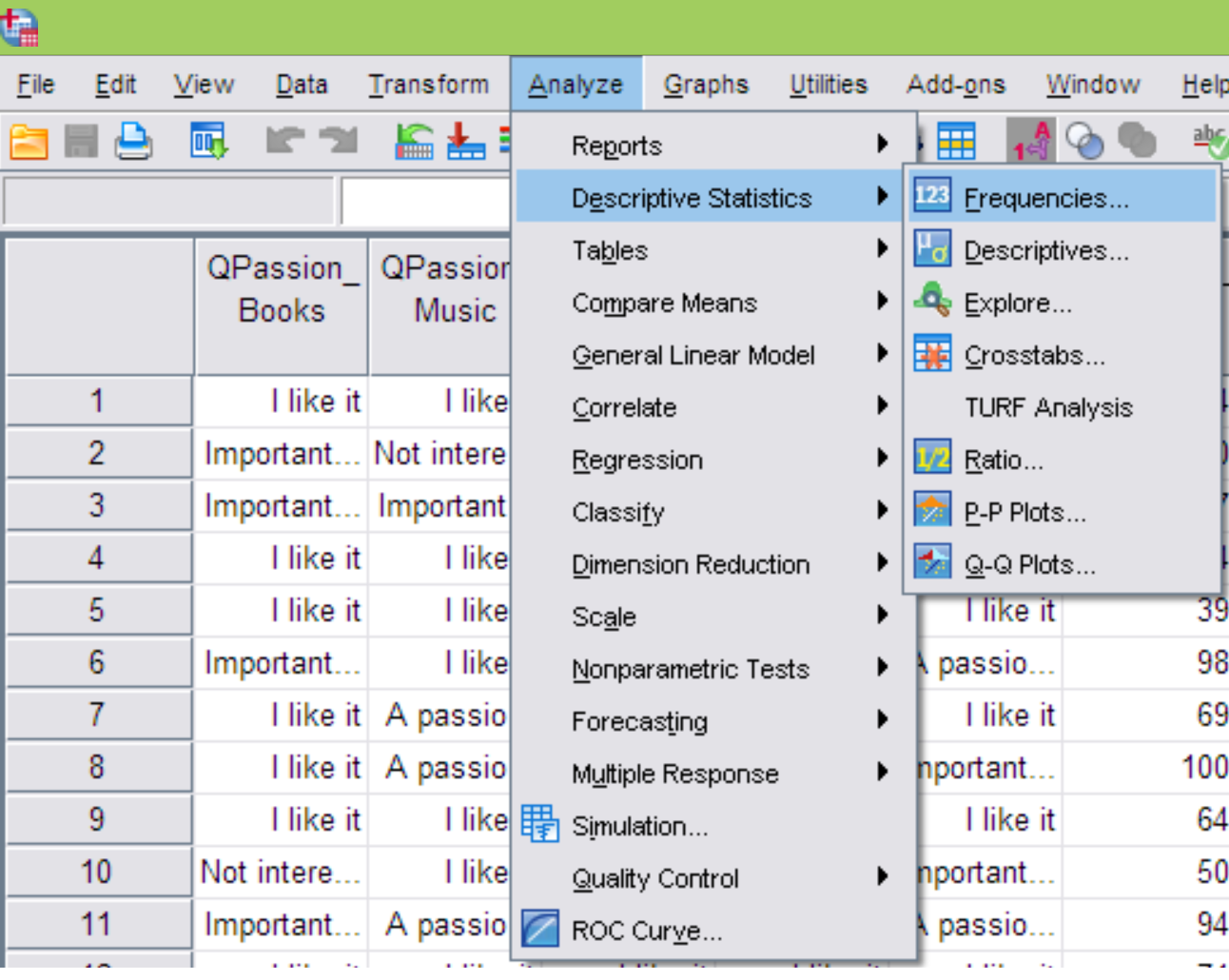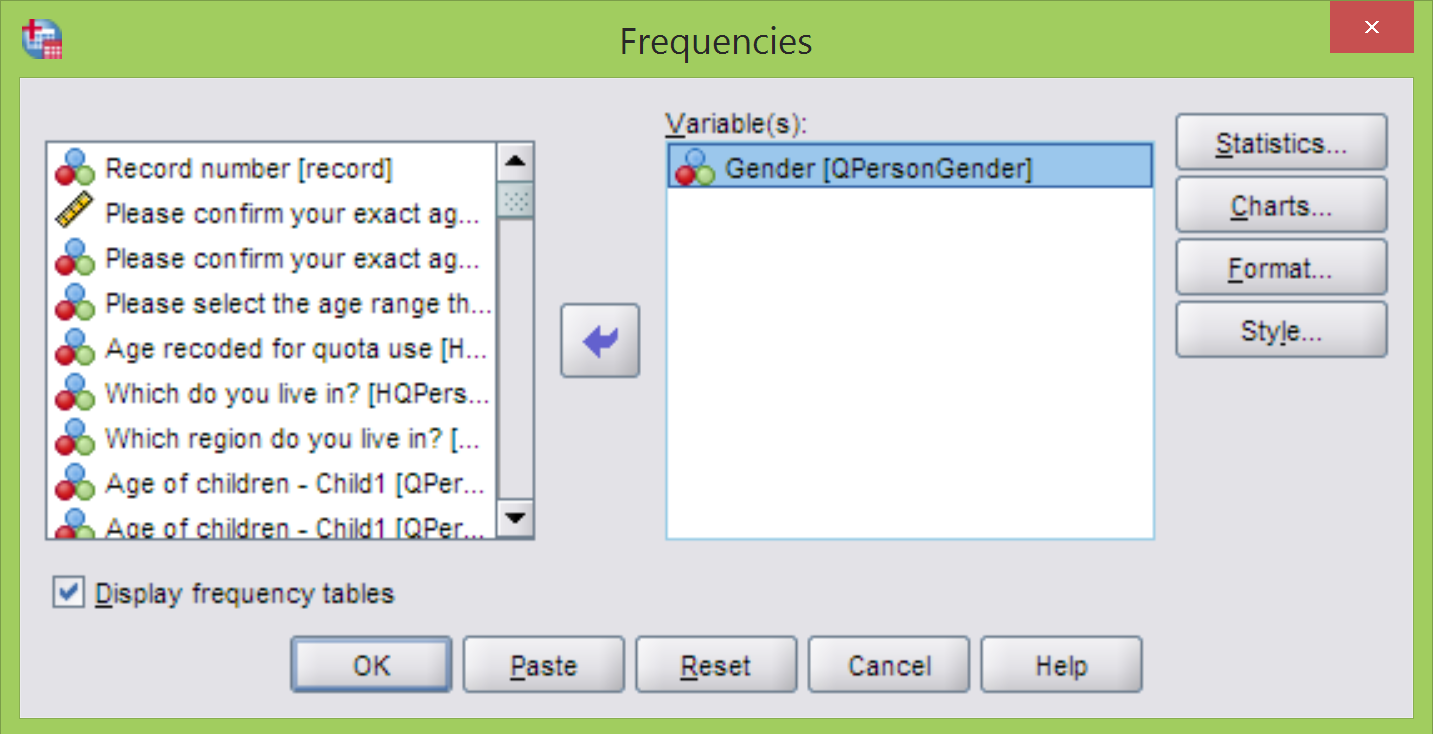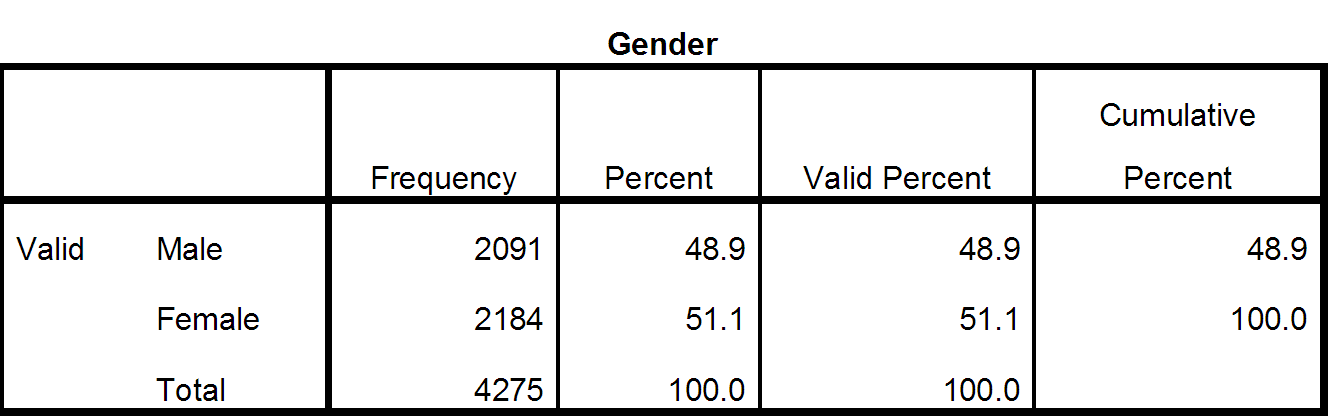# Getting to know your data

Getting to know your SPSS data

In this section:

·        Frequencies

·        Crosstabs

·        Weighting

Best way to familiarise yourself with the data is to run some basic statistics.

The simplest one of all is “Frequencies”. It gives you a simple read of one (or multiply) variables you select. By listing the occurrence (number of respondents) in each category of given variable. I.e. if you run frequencies on a Variable that records Gender – a single choice variable that can have two values: Male or Female – it will give you the number of Males and Females recorded in the data.

You can access the Frequencies command via the following path:

Analyze > Descriptive Statistics > Frequencies…From the Frequencies menu point you can simply select the variable(s) you are interested in, and move them (either by dragging them over to the right (“Variable(s)” field) or using the arrow icon in the middle:By clicking OK you’ll get the following simple table:It simply shows that there are 2,091 Males (48.9%) & 2,184 Females (51.1%) in your sample of 4,275.

In some cases, e.g. when you have a scale variable like “Exact age” – running frequencies would result in a table that potentially could have 100+ rows each of them with small occurrences. (I.e. in this dataset you’d have 1 person who is 88 years old, etc.). For this type of variables it worth to look into other statistics and omit the frequency tables by unselecting “Display frequency tables” in the bottom left corner, and select the statistics interested from the “Statistics” menu point: